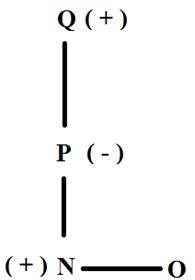# Crack SBI Clerk / Canara Bank PO 2018 – Logical Reasoning (Miscellaneous) Day-19

## Crack SBI Clerk / Canara Bank PO 2018 – Logical Reasoning (Miscellaneous) Day-19:

Dear Readers, Reasoning Section play a vital role in Banking and all other competitive exams. This year 2018 was started with three wonderful opportunities; Canara Bank PO Exam and Most Awaited SBI Clerk 2018. To enrich your preparation here we have providing new series of Practice Questions on Reasoning Ability – Miscellaneous. Candidates those who are going to appear in Bank Exams 2018 can practice these questions daily and make your preparation effective.

[WpProQuiz 1373]

## Daily Practice Test Schedule | Good Luck

 Topic Daily Publishing Time Daily News Papers & Editorials 8.00 AM Current Affairs Quiz 9.00 AM Logical Reasoning 10.00 AM Quantitative Aptitude “20-20” 11.00 AM Vocabulary (Based on The Hindu) 12.00 PM Static GK Quiz 1.00 PM English Language “20-20” 2.00 PM Banking Awareness Quiz 3.00 PM Reasoning Puzzles & Seating 4.00 PM Daily Current Affairs Updates 5.00 PM Data Interpretation / Application Sums (Topic Wise) 6.00 PM Reasoning Ability “20-20” 7.00 PM English Language (New Pattern Questions) 8.00 PM General / Financial Awareness Quiz 9.00 PM

Directions (1 – 5): In the following questions, the symbols %, @, #, \$ and & are used with the following meaning as illustrated below:

‘P%Q’ means ‘P is neither smaller than nor equal to Q’.

[email protected]’ means ‘P is neither greater than nor equal to Q’.

‘P#Q’ means ‘P is not greater than Q’.

‘P\$Q’ means ‘P is not smaller than Q’.

‘P&Q’ means ‘P is neither smaller than nor greater than Q’.

Now in each of the following questions, assuming the given statements to be true, find which of the three conclusions I, II, and III given below them is/are definitely true and give your answer accordingly.

1) Statements: A%S, S\$W, W&F, [email protected]

Conclusions:

III. X%W

a) Only I

b) Only II

c) Both II and III

d) All I, II and III

e) Only I and II

Answer c) Both II and III

% –  >

@ – <

# –  ≤

\$ –  ≥

& – =

A > S ≥ W = F < X,

I) S > F (False)

II) W < A (True)

III) X > W (True)

2) Statements: R&W, W#C, C%A, A\$V

Conclusions:

1. C&R
2. A%W

a) Only II

b) Only I

c) Only I and III

d) Only III

e) None of these

R = W ≤ C > A ≥ V

I) C = R (False)

II) A > W (False)

III) V < C (True)

3) Statements: [email protected], D\$M, M#S, S&J

Conclusions:

1. A\$M
2. J&D

a) Either I or III

b) Only I

c) Only II and III

d) All I, II and III

e) Either I or III and II

Answer a) Either I or III

A < D ≥ M ≤ S = J

I) A ≥ M (False)

II) J = D (False)

III) A < M (False)

So no relation between D and J and no relation between M and A

In I and III we see subject predicate same and since no relation and I is A ≥ M and III is A < M so either or

4) Statements: C#A, X%A, R\$X, [email protected]

Conclusions:

1. R%A
2. R\$A

a) Both I and II

b) Both I and III

c) Both II and III

d) Either I or II and III

e) Only I

Answer b) Both I and III

C ≤ A < X ≤ R < S

I) R > A (True)

II) R ≥ A (False)

III) C < S (True)

5) Statements: X\$E, E%W, W&Q, [email protected]

Conclusions:

III. E&A

a) Only III

b) Both I and II

c) All I, II and III

d) Both II and III

e) None of these

X ≥ E > W = Q < A

I) A < W (False)

II) X > Q (True)

III) E = A (False)

Only II follows

6) A man walks 5 km towards south and then turns to his right. After walking 3 km he turns to the left and walks 5 km. Now in which direction is he from the starting point?

a) West

b) South

c) North-East

d) South-West

e) None of these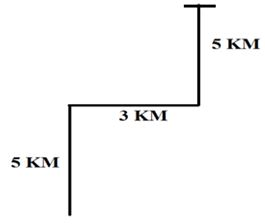7) Shakthi starts walking from a point towards west 5km, then turns right and walks 5km. Now at what distance she is from the starting point?

a) 15√2 km

b) 6√2 km

c) 8 √2 km

d) 5√2km

e) None of these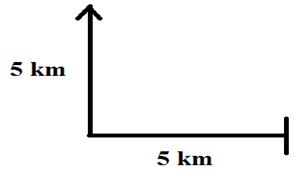8) How many such pairs of letters are there in the word ‘CHILDREN’ each of which has as many letters between them in the word as there are between them in the English alphabet(in both directions Left to right and right to left)?

a) 4

b) 5

c) 3

d) 1

e) None of these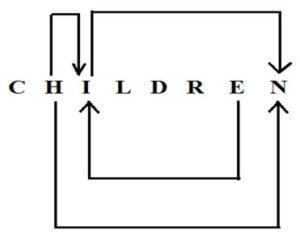Direction (9 – 10) Read the instructions and answer the questions given below

A + B means A is the mother of B

A – B means A is the brother B

A % B means A is the father of B

A x B means A is the sister of B

9) Which of the following shows that P is the maternal uncle of Q?

a) Q – N + M x P

b) P + S x N – Q

c) P – M + N x Q

d) Q – S % P

e) None of these

Answer c) P – M + N x Q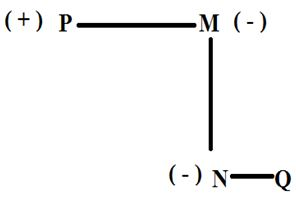10) Which of the following shows that Q is the maternal Grandfather of O?

a) Q – N % M x P

b) S x N – Q

c) P – M + N

d) Q % P + N – O

e) None of these

Answer d) Q % P + N – O